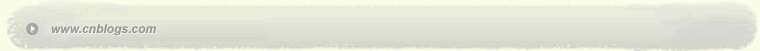C++分析研究 C++

 < 2023年2月 >
2930311234
567891011
12131415161718
19202122232425
2627281234
567891011

• 随笔 - 92
• 文章 - 4
• 评论 - 4
• 引用 - 0

# 文章档案

•

### 评论排行榜

基本的计算步骤

1. 计算出基本操作的执行次数T（n）

2. 计算出T（n）的数量级

3. 用大O来表示时间复杂度

（1） int num1, num2;
（2） for（int i=0; i<n; i++）{
（3） num1 += 1;
（4） for（int j=1; j<=n; j*=2）{
（5） num2 += num1;
（6） }
（7） }

1.

T（n） = 2 + 4n + 3n*log2n
2.

f（n） = n*log2n
3.
lim（T（n）/f（n）） = （2+4n+3n*log2n） / （n*log2n）
= 2*（1/n）*（1/log2n） + 4*（1/log2n） + 3

T（n） = O（n*log2n）

1. 找到执行次数最多的语句
2. 计算语句执行次数的数量级
3. 用大O来表示结果

1.

2.
T（n） = n*log2n
f（n） = n*log2n
3.
// lim（T（n）/f（n）） = 1
T（n） = O（n*log2n）
--------------------------------------------------------------------------------

--------------------------------------------------------------------------------

1） 加法规则
T（n,m） = T1（n） + T2（n） = O （max （ f（n）， g（m） ）
2） 乘法规则
T（n,m） = T1（n） * T2（m） = O （f（n） * g（m））
3） 一个特例（问题规模为常量的时间复杂度）

T（n） = T1（n） * T2（n） = O （ c*f（n） ） = O（ f（n） ）

4） 一个经验规则

c < log2n < n < n*log2n < n2 < n3 < 2n < 3n < n! （c是一个常量）
|--------------------------|--------------------------|-------------|

--------------------------------------------------------------------------------------------------

1.并列循环的复杂度分析

for （i=1; i<=n; i++）
x++;
for （i=1; i<=n; i++）
for （j=1; j<=n; j++）
x++;

T（n） = n
f（n） = n

T（n） = n2
f（n） = n2

2.函数调用的复杂度分析

public void printsum（int count）{
int sum = 1;
for（int i= 0; i<n; i++）{
sum += i;
}
System.out.print（sum）；
}

*这里其实可以运用公式 num = n*（n+1）/2,对算法进行优化，改为：
public void printsum（int count）{
int sum = 1;
sum = count * （count+1）/2;
System.out.print（sum）；
}

3.混合情况（多个方法调用与循环）的复杂度分析

public void suixiangMethod（int n）{
printsum（n）；//1.1
for（int i= 0; i<n; i++）{
printsum（n）； //1.2
}
for（int i= 0; i<n; i++）{
for（int k=0; k
System.out.print（i,k）； //1.3
}
}
suixiangMethod 方法的时间复杂度需要计算方法体的各个成员的复杂度。

--------------------------------------------------------------------------------------------------

O（1）

temp=i;
i=j;
j=temp;

O（n2）
sum=0; /* 执行次数1 */
for（i=1;i<=n;i++）
for（j=1;j<=n;j++）
sum++; /* 执行次数n2 */

for （i=1;i<n;i++）
{
y=y+1; ①
for （j=0;j<=（2*n）；j++）
x++; ②
}

T（n） = 2n2-n-1+（n-1） = 2n2-2
f（n） = n2
lim（T（n）/f（n）） = 2 + 2*（1/n2） = 2
T（n） = O（n2）。
O（n）
a=0;
b=1; ①
for （i=1;i<=n;i++） ②
{
s=a+b; ③
b=a; ④
a=s; ⑤
}

T（n） = 2+4n
f（n） = n
lim（T（n）/f（n）） = 2*（1/n） + 4 = 4
T（n） = O（n）。
O（log2n）
i=1; ①
while （i<=n）
i=i*2; ②

T（n） = 1 + log2n
f（n） = log2n
lim（T（n）/f（n）） = 1/log2n + 1 = 1
T（n） = O（log2n）
O（n3）
for（i=0;i<n;i++）
{
for（j=0;j<i;j++）
{
for（k=0;k<j;k++）
x=x+2;
}
}

T（n） = n（n+1）（n-1）/2 = （n3-n）/2
f（n） = n3

posted on 2013-11-06 07:25 HAOSOLA 阅读(3154) 评论(0)  编辑 收藏 引用Copyright © HAOSOLA Powered by: 博客园 模板提供：沪江博客